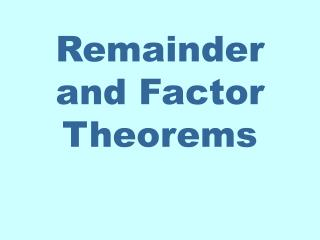DownloadDownload PresentationRemainder and Factor Theorems

# Remainder and Factor Theorems

Download Presentation## Remainder and Factor Theorems

- - - - - - - - - - - - - - - - - - - - - - - - - - - E N D - - - - - - - - - - - - - - - - - - - - - - - - - - -
##### Presentation Transcript

1. Remainder and Factor Theorems

2. REMAINDER THEOREM Let f be a polynomial function. If f (x) is divided by x – c, then the remainder is f (c). Let’s look at an example to see how this theorem is useful. So the remainder we get in synthetic division is the same as the answer we’d get if we put -2 in the function. The root of x + 2 = 0 is x = -2 using synthetic division let’s divide by x + 2 -2 2 -3 2 -1 -4 14 -32 2 -7 16 -33 the remainder Find f(-2)

3. FACTOR THEOREM Let f be a polynomial function. Then x – c is a factor of f (x) if and only if f (c) = 0 -3 -4 5 0 8 12 -51 153 -4 17 -51 161 If and only if means this will be true either way: 1. If f(c) = 0, then x - c is a factor of f(x) 2. If x - c is a factor of f(x) then f(c) = 0. Try synthetic division and see if the remainder is 0 Opposite sign goes here NO it’s not a factor. In fact, f(-3) = 161 We could have computed f(-3) at first to determine this. Not = 0 so not a factor

4. Acknowledgement I wish to thank Shawna Haider from Salt Lake Community College, Utah USA for her hard work in creating this PowerPoint. www.slcc.edu Shawna has kindly given permission for this resource to be downloaded from www.mathxtc.com and for it to be modified to suit the Western Australian Mathematics Curriculum. Stephen Corcoran Head of Mathematics St Stephen’s School – Carramar www.ststephens.wa.edu.au